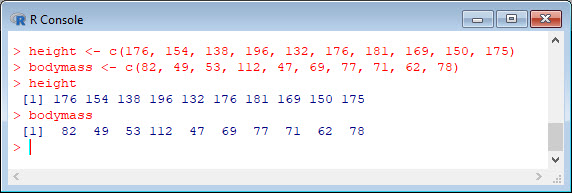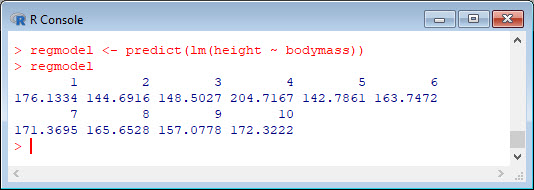In my last blog we created two variables and used the `lm()` command to perform a least squares regression on them, treating one of them as the dependent variable and the other as the independent variable. Here they are again.
```height = c(176, 154, 138, 196, 132, 176, 181, 169, 150, 175) bodymass = c(82, 49, 53, 112, 47, 69, 77, 71, 62, 78)```
Today we learn how to obtain useful diagnostic information about a regression model and then how to draw residuals on a plot. As before, we perform the regression.
`lm(height ~ bodymass)`Now let’s find out more about the regression. First, let’s store the regression model as an object called `mod` and then use the `summary()` command to learn about the regression.
`mod <- lm(height ~ bodymass)`
`summary(mod)`
Here is what R gives you.R has given you a great deal of diagnostic information about the regression. The most useful of this information are the coefficients themselves, the Adjusted R-squared, the F-statistic and the p-value for the model.
Now let’s use R’s `predict()` command to create a vector of fitted values.
`regmodel <- predict(lm(height ~ bodymass))`
`regmodel`
Here are the fitted values:Now let’s plot the data and regression line again.
```plot(bodymass, height, pch = 16, cex = 1.3, col = "blue", main = "HEIGHT PLOTTED AGAINST BODY MASS", xlab = "BODY MASS (kg)", ylab = "HEIGHT (cm)") abline(lm(height ~ bodymass))```We can plot the residuals using R’s for loop and a subscript `k` that runs from 1 to the number of data points. We know that there are 10 data points, but if we do not know the number of data we can find it using the `length()` command on either the height or body mass variable.
`npoints <- length(height)`
`npoints`Now let’s implement the loop and draw the residuals (the differences between the observed data and the corresponding fitted values) using the `lines()` command. Note the syntax we use to draw in the residuals.
`for (k in 1: npoints) lines(c(bodymass[k], bodymass[k]), c(height[k], regmodel[k]))`
Here is our plot, including the residuals.None of this was so difficult!
Next time we will look at more advanced aspects of regression models and see what R has to offer. See you then!
David

#### Annex: R codes used

```# Create two variables.
height = c(176, 154, 138, 196, 132, 176, 181, 169, 150, 175)
bodymass = c(82, 49, 53, 112, 47, 69, 77, 71, 62, 78)

# Estimate the regression model.
lm(height ~ bodymass)

# Store the regression model as an object.
mod <- lm(height ~ bodymass)
summary(mod)

# Create a vector of fitted values.
regmodel <- predict(lm(height ~ bodymass))
regmodel

# Plot the data and regression line.
plot(bodymass, height, pch = 16, cex = 1.3, col = "blue", main = "HEIGHT PLOTTED AGAINST BODY MASS", xlab = "BODY MASS (kg)", ylab = "HEIGHT (cm)")
abline(lm(height ~ bodymass))

# Find the number of data.
npoints <- length(height)
npoints

# Draw in the residuals.
for (k in 1: npoints) lines(c(bodymass[k], bodymass[k]), c(height[k], regmodel[k]))
```